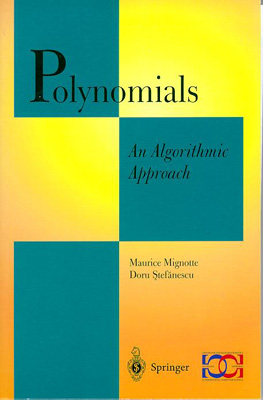M. Mignotte, D. Stefanescu. Polynomials. An Algorithmic Approach, Springer-Verlag, Singapore, 1999. Approx. 320pp. ISBN: 981-4021-51-2. US\$49 softcover.

This textbook gives a well-balanced presentation of the classic procedures of polynomial algebra which are computationally relevant and some algorithms developed during the last decade. The first chapter discusses the constrcution and the representation of polynomials. The second chapter focuses on the computational aspects of the analytical theory of polynomials. Polynomials with coefficients in a finaite field are then described in chapetr three, and the final chapter is devoted to factorization of polynomials with integral coefficients.

The book is primarily aimed at graduate students taking courses in Polynomial Algebra, with a prerequisite knowledge of set theory, usual fields and basic algebra. Fully worked out examples, hints and references complement the main text, and details concerning the implementation of algorithms as well as indicators of their efficiency are provided. The book is also useful as a supplementary text for courses in scientific computing, analysis of algorithms, computational polynomial factorization, and computational geometry of polynomials.

Contents: 1. An Introduction to Polynomials: Construction and representation of polynomials; Complexity and cost; Polynomial division; Polynomial factorization; Polynomial roots. Eliminations. Resultants; Symmetric functions; Polynomial interpolation; Irreducinility criteria. 2. Complex Polynomials: Polynomial size; Geometry of polynomials; Stable polynomials; Polynomial roots inside the unit disk; Bounds for the roots; Applications to integer polynomials; Separation of roots. 3. Polynomials with Coefficients in a Finite Field: Finite fields; Cyclotomic polynomials; Fast Fourier transform; Number of irreducible polynomials over a finite field; Constrcution of irreducible polynomials over a finite field; Roots of polynomials over finite fields; Squarefree polynomials; Berlekamp's algorithm; Niederreiter's algorithm. 4. Integer Polynomials: Kronecker's factorization method; The berlekamp-Zassenhaus algorithm; The LLL factorization algorithm. Bibliography; Notation; List of Algorithms; Index.

Reviews:

• Letter by H. Niederreiter
• MR: 2000e:12001 The aim of the book is to give an introduction to some polynomial theories with particular attention to the algorithmic aspect of the problems. All the results presented regard polynomials in one indeterminate. The book is divided into four chapters, as follows. The first chapter introduces some basic notions, including complexity of polynomial operations, polynomial division, resultants and elimination theory, interpolations and some irreducibility criteria. Chapter two considers complex polynomials and is essentially dedicated to presenting several techniques to bound complex roots and to isolate them inside (or outside) disks of the complex plane. The third chapter introduces the theory of polynomials over finite fields and contains the definition and some properties of cyclotomic polynomials, as well as discrete/discrete fast Fourier transform and construction of irreducible polynomials over a finite field. Successively in this chapter the authors also explain the Berlekamp and Niederreiter algorithms for factorization of polynomials with coefficients in finite fields \$Z\sb p\$. Finally, the last chapter deals with the problem of factorization of polynomials with integer coefficients. In particular the authors present the Berlekamp-Zassenhaus algorithm and the LLL factorization algorithm. This textbook is addressed to graduate students in mathematics and computer science who want to improve their knowledge in the field of polynomial theories and computational algebra. Several topics of the book take advantage of very recently developed techniques and in this sense the book can be a good source for the state of the art in the subjects it presents. Each section of the book is integrated with several exercises with different levels of difficulty. Reviewed by Alessandro Logar
• ZbL: This book is devoted to the study of polynomials and includes several relevant algorithms (some of them classic, others more recent) with detailed analysis of their algebraic complexity. The book is structured in four chapters. \par The first begins with the formal definition of the ring of polynomials and with two different ways of representing polynomials: dense and sparse form. There is a study of the complexity of polynomial operations using both ways of coding. A few basic definitions and facts on polynomials are exposed (divisibility, Euclidean algorithm, gcd, factorization, roots). Some notions of elimination theory (resultant, Bezoutian) and of polynomial interpolation are given. The chapter ends with some irreducibility criteria in one and several variables. \par The second chapter is based in the analytic theory of complex polynomials: the authors define different polynomial sizes (norm, Mahler measure, length and height) and give upper bounds for factors of a given polynomial. Then, many interesting results on location of roots in the complex plane are shown and finally these results are applied to integer polynomials. In the third chapter, they present the theory of finite fields and cyclotomic polynomials in order to show how the discrete fast Fourier transform gives a fast algorithm to multiply polynomials. After some results on irreducible polynomials over finite fields, both Berlekamp's algorithm and Niederreiter's algorithm for factorization of polynomials over finite fields are thoroughly discussed. In the last chapter, three algorithms to give the factorization of polynomials over the ring of integers are described: Kronecker's factorization method, the Berlekamp-Zassenhaus algorithm and the Lenstra-Lenstra-Lovasz (LLL) algorithm which shows that factorization of polynomials over the ring of integers can be performed in polynomial time. The book is almost self-contained and the exposition is clear but a certain background in algebra is needed. There are many interesting exercises at the end of each section, some of them extracted of recent research papers. Reviewed by Juan Sabia